# Elimination of grammar left recursion in Python

2022-02-02 00:59:32

## Preface

1. After lexical analysis , Then comes the category of grammatical analysis . Several sub problems need to be solved to complete syntax analysis , Complete the elimination of grammar left recursion today .
2. Didn't learn from any blogs , Make your own wheels .

## Before the start

1. The core of grammar left recursion elimination program is the processing of string , Enter the production as a string , Split it 、 Replace and merge operations run through , The logic and thinking of the processing process will be full of loopholes if there are slight mistakes and omissions .
2. use Direct rewriting method , It's hard to read the code without understanding the left recursive elimination method .

## requirement

1. CFG Grammar judgment
2. Left recursive type
3. Left recursion and indirect recursion
4. Interface

## Source code

``````import os
import tkinter as tk
import tkinter.messagebox
import tkinter.font as tf

zhuizhong = ""
wenfa = {" Non left recursive grammar "}
xi_ = ""
huo = ""

window = tk.Tk()
window.title(' Eliminate left recursion ')
window.minsize(500,500)
# The converted coordinates are displayed as tuples
def getIndex(text, pos):
return tuple(map(int, str.split(text.index(pos), ".")))

def zhijie(x,y):
if not len(y):
pass
else:
if x == y:
wenfa.discard(" Non left recursive grammar ")
# Handle direct left recursion
zuobian = y.split('|')
feizhongjie = []
zhongjie = []
for item in zuobian:
if x in item:
item = item[1:]
textt = str(item) + str(x) + "'"
feizhongjie.append(textt)
else:
text = str(item) + str(x) + "'"
zhongjie.append(text)
if not zhongjie:# Handle A -> Ax The situation of
zhongjie.append(str(x + "'"))
cheng = str(x) + " -> " + "|".join(zhongjie)
zi = str(x) + "'" + " -> " + "|".join(feizhongjie) + "|є"
text_output.insert('insert',' Direct left recursive grammar ','tag1')
text_output.insert('insert','\n')
text_output.insert('insert',cheng,'tag2')
text_output.insert('insert','\n')
text_output.insert('insert',zi,'tag2')
'''  In addition, it will judge the output of non recursive production , However, it will lead to indirect left recursion and cannot delete redundant production  else: h =" unchanged ： " + x + " -> " + y text_output.insert('insert',' Non left recursive grammar ','tag1') text_output.insert('insert','\n') text_output.insert('insert',h,'tag2') '''
text_output.insert('insert','\n')

def zhijie2(x,y):
if not len(y):
pass
else:
if x == y:
wenfa.discard(" Non left recursive grammar ")
# Handle direct left recursion
zuobian = y.split('|')
feizhongjie = []
zhongjie = []
for item in zuobian:
if x in item:
item = item[1:]
textt = str(item) + str(x) + "'"
feizhongjie.append(textt)
else:
text = str(item) + str(x) + "'"
zhongjie.append(text)
cheng = str(x) + " -> " + "|".join(zhongjie)
zi = str(x) + "'" + " -> " + "|".join(feizhongjie) + "|є"
text_output.insert('insert'," Indirect left recursive grammar ",'tag1')
text_output.insert('insert','\n')
text_output.insert('insert',cheng,'tag2')
text_output.insert('insert','\n')
text_output.insert('insert',zi,'tag2')

text_output.insert('insert','\n')

def tihuan(xk,yi,yk):
yi_you = []
yi_wu =[]
yi_he = ""
yi_wuhe = ""
yi_zhong = ""
yi_feizhong = []
if xk in yi:
yk_replace = yk.split('|')
yi_fenjie = yi.split('|')# Separate non terminality from non terminality
for ba in yi_fenjie:
if xk in ba:
yi_you.append(ba)
else:
yi_wu.append(ba)

yi_he = "|".join(yi_you)

for item in yk_replace:
yi_zhong = yi_he.replace(xk,item)# Replace
yi_feizhong.append(yi_zhong)
yi_wuhe = "|".join(yi_wu)# Re merger
global zhuizhong
zhuizhong = "|".join(yi_feizhong) + "|" + yi_wuhe

# The function executed after clicking the button
def changeString():
text_output.delete('1.0','end')
text = text_input.get('1.0','end')
text_list = list(text.split('\n'))# Line by line grammar
text_list.pop()
if not text_list:
print(tkinter.messagebox.showerror(title = ' Something went wrong ！',message=' Input cannot be empty '))
else:
for cfg in text_list:

x,y = cfg.split('->')# Separate grammar from left and right
x = ''.join(x.split())# Eliminate spaces
y = ''.join(y.split())
if not (len(x) == 1 and x >= 'A' and x <= 'Z'):
pos = text_input.search(x, '1.0', stopindex="end")
result = tkinter.messagebox.showerror(title = ' Something went wrong ！',
message=' Non context free grammar ! coordinate %s'%(getIndex(text_input, pos),))
#  The return value is ：ok
print(result)
return 0
else:
zhijie(x,y)

for i in range(len(text_list)):
for k in range(i):
xi,yi = text_list[i].split('->')
xi = ''.join(xi.split())# Eliminate spaces
yi = ''.join(yi.split())

xk,yk = text_list[k].split('->')
xk = ''.join(xk.split())# Eliminate spaces
yk = ''.join(yk.split())

tihuan(xk,yi,yk)
tihuan(xk,zhuizhong,yk)
global xi_
xi_ = xi
zhijie2(xi_,zhuizhong)

for item in wenfa:
text_output.insert('insert',item,'tag1')

# Create text input boxes and buttons
text_input  = tk.Text(window, width=80, height=16)
text_output = tk.Text(window, width=80, height=20)
# Simple style
ft = tf.Font(family=' Microsoft YaHei ',size=12)
text_output.tag_config("tag1",background="yellow",foreground="red",font=ft)
text_output.tag_config('tag2',font = ft)
# Button
button = tk.Button(window,text=" Eliminate left recursion ",command=changeString,padx=32,pady=4,bd=4)

text_input.pack()
text_output.pack()
button.pack()
window.mainloop()
Copy code ``````

## Is it difficult to understand , Take a look at the flow chart of half hanging son

• Main process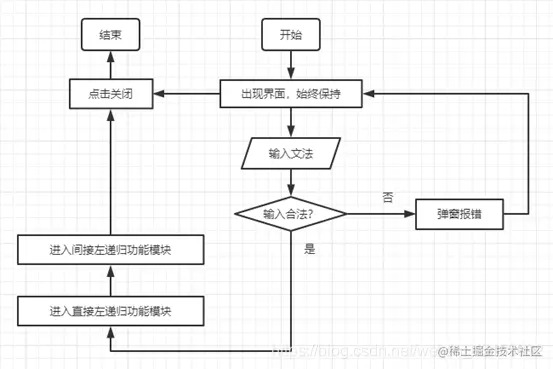• Direct left recursion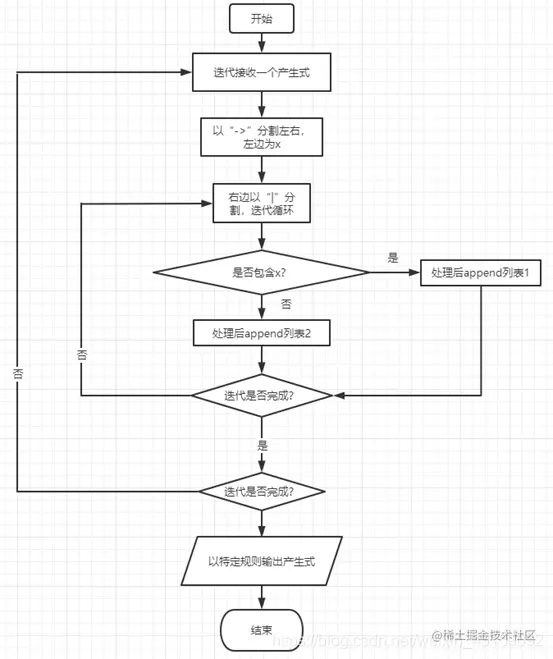• Indirect left recursive merge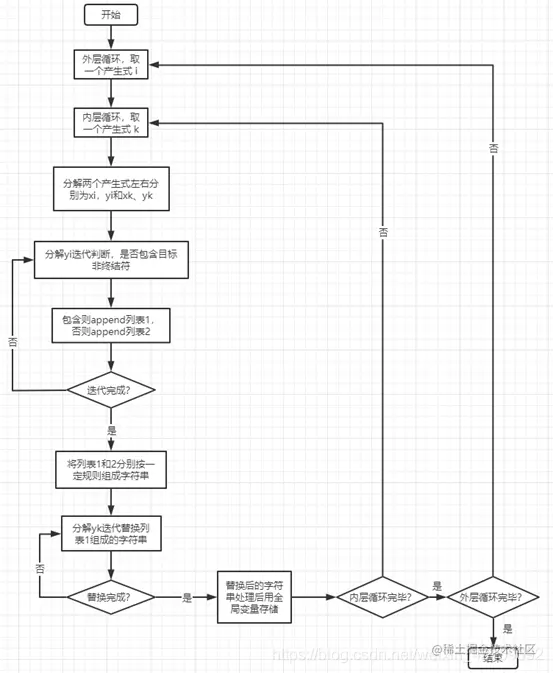## Run a screenshot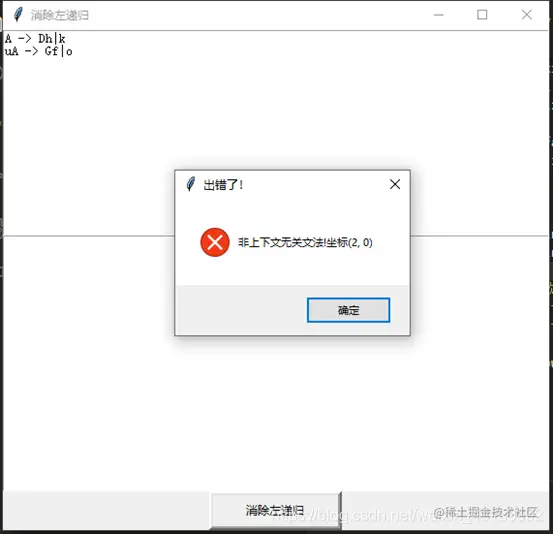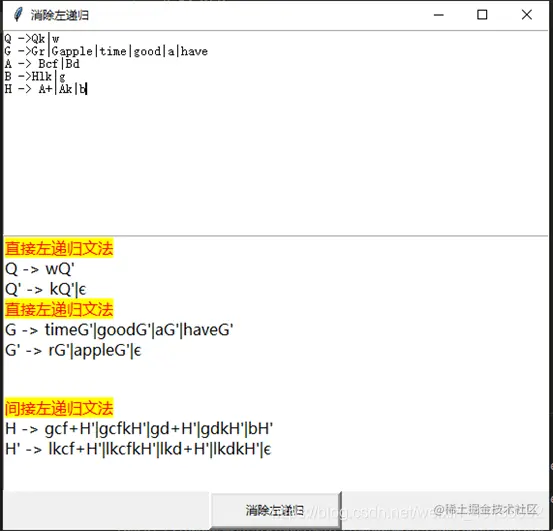## summary

（1） Orient

It's not hard to do one thing , The hardest thing is that there is no direction , I don't know what to do ; I just feel that time goes by, but I don't produce anything . Fortunately, there are specific topics to choose from , This time, after a little entanglement , Decisively choose grammar left recursion to eliminate , Tell the truth , I think this is the simplest .

（2） Start implementation

First, understand the method of eliminating left recursion thoroughly , Found that the essence of the program is the operation of strings . Complete the direct left recursive algorithm very smoothly , I am rigorous and step by step , Hardly any bug, The follow-up test only adds the judgment of some edge conditions , For example, null value , Let the program face complex production easily . The algorithm of merging the production of indirect left recursion is also very smooth , Because I have outlined on the draft paper what I need to get at each step , When writing code, , One output at a time , See if it meets expectations , Subsequent tests slightly improved robustness . The real difficulty lies in the idea , Even the outermost two iterations have been considered for a long time . The logic and thinking of these two algorithms are very complex , The opening and closing of strings , Store separately , Use no less than ten list and string data types , Plus a few global variables , I'm a little proud of my clear thinking .

（3） deficiencies

1、 I hope to achieve , Non left recursive grammar , The input of left recursion and indirect left recursion is recognized and eliminated together , When you encounter a non left recursive grammar, you output “ Non left recursive grammar ”, Then output it without any modification . If you implement this , How to make indirect left recursion not be treated as non left recursion grammar ？ I didn't think of a solution . 2、 My judgment of non terminator is whether it contains , No further judgment of location , For example, eliminate D -> Dh|sD|h,D stay s after , This can't be handled well . 3、 The input order of indirect left recursive grammar production is required , I haven't been able to input freely yet .

（4） Problems encountered

The problems I encounter are all about the overall structure and trade-offs , For example, I finally chose to use two loops for input , One is to iterate over one production , Eliminate direct left recursion , The second one combines indirect left recursion with subscript nested two-level loops from the beginning . In addressing deficiencies 1 when , I spent a lot of time , Tried everything , For example, global variables , aggregate , Even back up the code , Make major changes , In the end, they compromised . When writing two core algorithms , What data type do I get at each step , Get what , Are very careful to confirm , Step by step , Didn't show up “bug Find a day ” The situation of . Each step requires a new variable storage , I'll add one at the beginning of the method ,tihuan() This method has six variables , Now that I think about it , The space complexity is very high .

（5） summary

This design is completely independent , No reference to any blog , I also know that some things that I think are very difficult are not worth mentioning in front of Daniel , Perhaps the overall architecture of the program is far from . in any case , I did what the title required , And it doesn't take long , I still have a sense of achievement . however , I will never be proud , There is no proud capital . Draw the interface from , Receive text input , Take the production , Judgment type , Eliminate direct left recursion , Merge indirect left recursion and then eliminate indirect left recursion . properly and logically arranged , One step at a time , Only by doing so can the building rise from the ground .